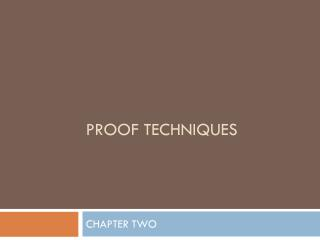Download PresentationProof techniques

# Proof techniques - PowerPoint PPT PresentationDownload Presentation## Proof techniques

- - - - - - - - - - - - - - - - - - - - - - - - - - - E N D - - - - - - - - - - - - - - - - - - - - - - - - - - -
##### Presentation Transcript

1. Proof techniques CHAPTER TWO

2. Formal Logic vs. Real-world Arguments • Real-world arguments, unlike the formal proofs of Chapter 1, are normally dependent on context, not the structure of the argument. • In other words, a real-world argument may not be universally valid, though it be valid in some important context. • Terminology: What is a conjecture? CSC 333

3. Argument Context • In real-world situations, we often are interested only in the truth of an argument in a particular context. • Example:“If Mary Beth (or some other student) makes an A in CSC 333, then she must be a bright, hard worker.” • Call this the Mary Beth Theorem. CSC 333

4. Examination of the Mary Beth Theorem • Can we state the M.B. Theorem formally? • Yes. Let “Mary Beth makes an A in CSC 333” be proposition P, and “Mary Beth is a bright, hard worker” be Q. • We can state the M.B. Theorem as P -> Q. • (Or perhaps more properly, let R be “Mary Beth is bright”, and let S be “Mary Beth is a hard worker”; thus, Q can be decomposed as R ^ S • So, we can state the M.B. Theorem as P-> (R ^ S). • We can easily establish a truth table for this. • As stated in the text, if we can’t translate a real-world argument into a formal proof, we should look askance at the argument. CSC 333

5. Attacking the M.B. Theorem • Disproving by counterexample: • Assume that Mary Beth can be shown to be an imbecile, although she has an A in CSC 333. • This would be a case where R (Mary Beth is bright) is false, making (R ^ S) false. • So, in at least one case P does NOT imply Q. CSC 333

6. Which is easier? • Proving a conjecture using a formal proof, i.e., showing that for all truth values of the propositions, the theorem holds. OR • Disproving a conjecture by showing one instance in which the theorem “folds” (does not hold), i.e., a counterexample. • Note that showing one example in which it holds is insufficient as a proof. • Aside: How does this apply to software testing? CSC 333

7. Exhaustive Proofs • If we have a finite population to which we are applying the M.B. Theorem, • say, the students in CSC 333 in spring of 2010, • And we can show the truth of the M.B. Theorem for all those students, • then we have proved the M.B. Theorem by exhaustion. • Aside: How does this apply to software testing? CSC 333

8. Direct Proof • If we want to prove the M.B. Theorem for all students who ever enroll in CSC 333, we might attempt a direct proof: • Assume P is true. • Show that the conjecture is universally true because R ^ S inevitably follows from P. • For the M.B. Theorem, we can’t show this. • See text for an example of such a proof (p. 92). CSC 333

9. Contraposition • Proving by contraposition: • We already know that P -> Q is logically equivalent to ~Q -> ~P. • So, if we prove, for example, that if Mary Beth is NOT both bright [R] and a hard worker [S], then Mary Beth will NOT get an A in CSC 333, we have proved the original conjecture. • Note that the if the contrapositive can be shown to be false in at least one case, this disproves the original conjecture. • What if Mary Beth is lazy but cheats cleverly? CSC 333

10. Contradiction • Proving by contradiction: • Show that P is true and Q is false is a contradiction, i.e., it is always false. • In other words, show that it cannot ever be true that P is true and Q is false. • See example 10, p. 95. • Proving that something is not true is usually more difficult than assuming it is true and then showing a contradiction. CSC 333

11. Terms • Conjecture • Inductive reasoning • Deductive reasoning • Counterexample • Direct proof • Contraposition • Contradiction CSC 333